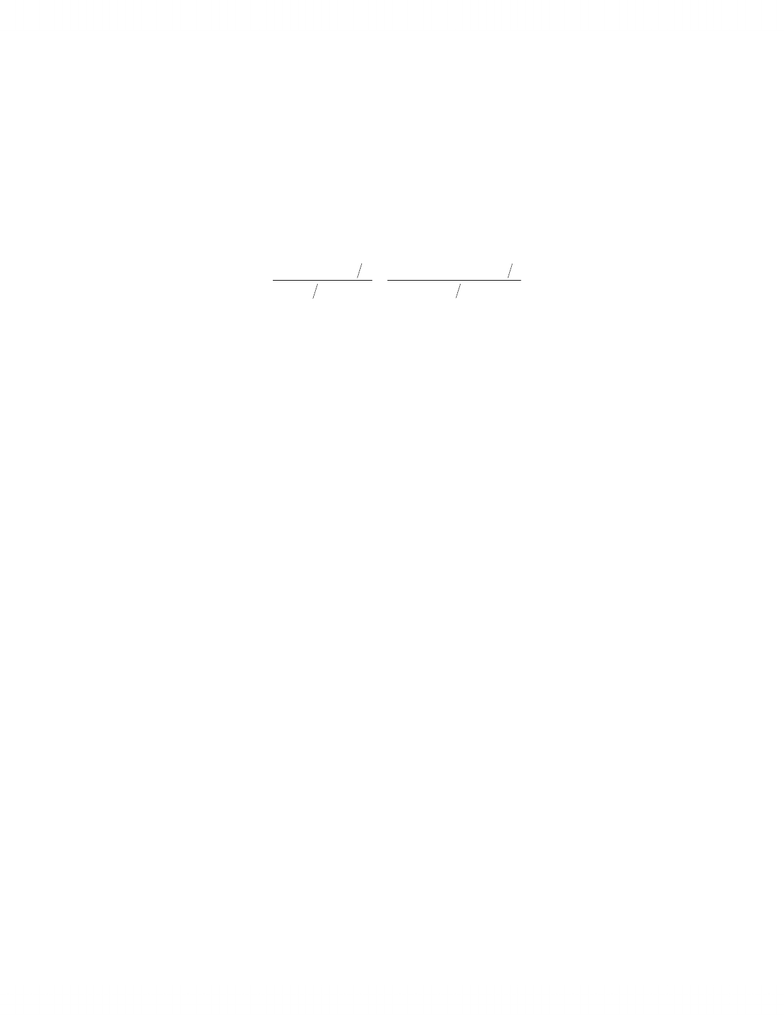# ECON 3210 Chapter Notes - Chapter 6: Econometrics, Null Hypothesis, Explained Variation

88 views12 pages
School
Department
Course
Professor178
CHAPTER 6
Exercise Solutions
Unlock document

This preview shows pages 1-3 of the document.
Unlock all 12 pages and 3 million more documents.Chapter 6, Exercise Solutions, Principles of Econometrics, 4e 179
EXERCISE 6.1
(a) To compute
2
R
, we need SSE and SST. We are given SSE. We can find SST from the
equation
2
()
ˆ13.45222
11
i
yyy SST
NN
  

Solving this equation for SST yields
22
ˆ( 1) (13.45222) 39 7057.5267
y
SST N 
Thus,
2979.830
1 1 0.8612
7057.5267
SSE
 
(b) The F-statistic for testing 02 3
:0H
  is defined as
( ) ( 1) (7057.5267 979.830) / 2 114.75
( ) 979.830/(40 3)
SST SSE K
FSSE N K
 
 

At
0.05 , the critical value is (0.95,2,37) 3.25F
. Since the calculated F is greater than
the critical F, we reject 0
H. There is evidence from the data to suggest that 20 and/or
30 .
Unlock document

This preview shows pages 1-3 of the document.
Unlock all 12 pages and 3 million more documents.Chapter 6, Exercise Solutions, Principles of Econometrics, 4e 180
EXERCISE 6.2
The model from Exercise 6.1 is 12 3
yxze
 . The SSE from estimating this
model is 979.830. The model after augmenting with the squares and the cubes of
predictions 23
ˆˆ
and yy
is 23
12 3 1 2
ˆˆ
yxzyye
 . The SSE from estimating
this model is 696.5375. To use the RESET, we set the null hypothesis 01 2
:0H.
The F-value for testing this hypothesis is
()
(979.830 696.5375) 2 7.1175
( ) 696.5373 (40 5)
RU
U
SSE SSE J
FSSE N K
 

The critical value for significance level 0.05
is (0.95,2,35) 3.267F
. Since the
calculated F is greater than the critical F we reject 0
H and conclude that the model is
misspecified.
Unlock document

This preview shows pages 1-3 of the document.
Unlock all 12 pages and 3 million more documents.

# Get access

\$10 USD/m
Billed \$120 USD annually
Homework Help
Class Notes
Textbook Notes